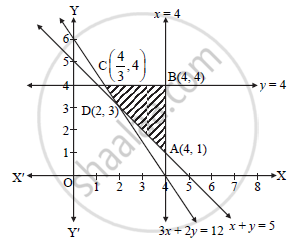HSC Science (General) 12th Board ExamMaharashtra State Board
Share

# Minimize : Z = 6x + 4y Subject to the conditions: 3x + 2y ≥ 12, x + y ≥ 5, 0 ≤ x ≤ 4, 0 ≤ y ≤ 4 - HSC Science (General) 12th Board Exam - Mathematics and Statistics

ConceptGraphical Method of Solving Linear Programming Problems

#### Question

Minimize : Z = 6x + 4y

Subject to the conditions:

3x + 2y ≥ 12,

x + y ≥ 5,

0 ≤ x ≤ 4,

0 ≤ y ≤ 4

#### Solution

To draw feasible region, construct table as follows:

 Inequality 3x+2y ≥12 x+y ≥ 5 x ≤ 4 y ≤ 4 Correspondingequation (of line) 3x + 2y = 12 x+y=5 x=4 y=4 Intersection of linewith X-axis (4, 0) (5, 0) (4, 0) - Intersection of linewith Y-axis (0, 6) (0, 5) - (0, 4) Region Non-origin side Non-origin side Origin side Origin sideShaded portion ABCD is the feasible region, whose vertices are A, B, C and D.
A is the point of intersection of the lines x = 4 and x + y = 5.
Putting x = 4 in x + y = 5, we get
4 + y = 5
y = 1
A = (4, 1)
B is the point of intersection of the lines y = 4 and x = 4.
B = (4, 4)
C is the point of intersection of the lines y = 4 and 3x + 2y = 12.
Putting y = 4 in 3x + 2y = 12, we get
3x + 2(4) = 12

x = 4/3

C≡(4/3,3 )

D is the point of intersection of the lines x + y = 5 and 3x + 2y = 12.
Solving the above equations, we get

D≡(2,3)

A≡(4,1),B≡(4,4),C≡(4/3,3 ) and D≡(2,3)

Here, the objective function is
Z = 6x + 4y
Z at A(4,1) = 6(4) + 4(1) = 28
Z at B(4, 4) = 6(4) + 4(4) = 40
Z at C (4/3 ,4)=6(4/3)+ 4(4) = 24
Z at D(2, 3) = 6(2) + 4(3) = 24

Thus, Z is minimized at every point along the line segment CD and its minimum value is 24.
Therefore, Z has infinite number of optimal solutions.

Is there an error in this question or solution?

#### APPEARS IN

2014-2015 (October) (with solutions)
Question 3.2.2 | 4.00 marks

#### Video TutorialsVIEW ALL 

Solution Minimize : Z = 6x + 4y Subject to the conditions: 3x + 2y ≥ 12, x + y ≥ 5, 0 ≤ x ≤ 4, 0 ≤ y ≤ 4 Concept: Graphical Method of Solving Linear Programming Problems.
S